Related Articles

# Create non-hierarchical columns with Pandas Group by module

• Last Updated : 16 Nov, 2020

In this article, we are going to see a couple of methods to create non-hierarchical columns when applying the groupby module.

We are using the Fortune 500 companies dataset to demonstrate the problem and solution. We have to grab a copy from data.world website.

For each “Sector” and “Industry” Find the total, average employees, and the minimum, maximum revenue change.

Let’s see an example with implementation:

Step 1: Let us begin by importing pandas and the data set with the company “Rank” as the index.

## Python3

 `import` `pandas as pd`` ` `# load the dataset``df ``=` `pd.read_csv(``    ``"https://raw.githubusercontent.com/sasankac/TestDataSet/master/Fortune500.csv"``)`` ` `# print the columns``print``(df.columns)`

Output: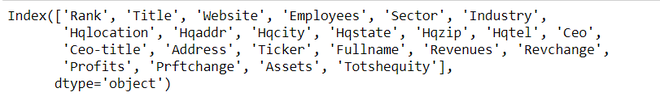Step 2: There are quite a number of columns that are of no interest to us in the dataset like Headquarters location, Address,…  I will be dropping them from the data set.

## Python3

 `# remove unwanted columns ``remove_columns ``=``[``'Website'``,``'Hqaddr'``,``'Hqzip'``, ``'Hqtel'``,``                 ``'Ceo'``,``'Ceo-title'``, ``'Address'``, ``'Ticker'``,``                 ``'Prftchange'``, ``'Assets'``, ``'Totshequity'``]`` ` `df ``=` `df.drop(columns``=` `remove_columns,axis ``=` `1``)``print``(df.columns)`

Output: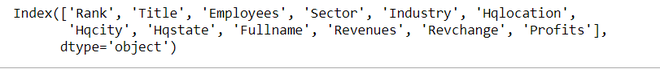Method 1:

In this method, we will be using to_flat_index method to output non-hierarchical columns. Let me, first group, the columns to identify the data for below. For each “Sector” and “Industry” Find the total, average employees, and the minimum, maximum revenue change.  The syntax for the groupby and aggregation is as follows

Syntax: df.groupby([‘grouping column1′,’ grouping column2”]).agg({ ‘aggregate column1’ :[‘aggregate function1′,’aggregate function2’] })

Now as per the requirement let us map the column names from the data set to the syntax.

• Grouping columns – ‘Sector’, ‘Industry’
• Aggregate columns – ‘Employees’, ‘Revchange’
• Aggregate functions – ‘sum’, ‘mean’, ‘min’ ”max3.   Getting the results by applying the above syntax.

Implementation:

## Python3

 `df_result ``=` `(df``           ``.groupby([``'Sector'``,``'Industry'``])``           ``.agg({``'Employees'``:[``'sum'``, ``'mean'``],``                 ``'Revchange'``:[``'min'``,``'max'``]}))`` ` `# printing top 15 rows``df_result.head(``15``)`

Output: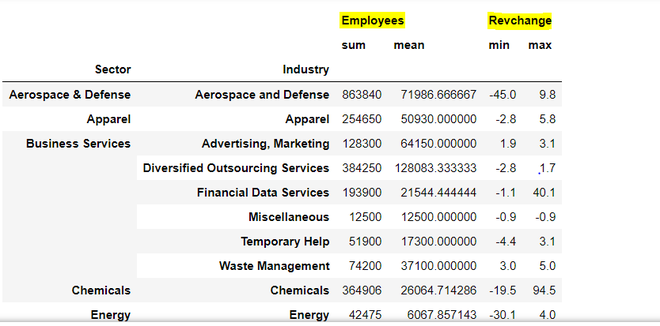Looking at the results, we have 6 hierarchical columns i.e. sum and mean for Employees (highlighted in yellow) and min, max columns for Revchange. We can convert the hierarchical columns to non-hierarchical columns using the .to_flat_index method which was introduced in the pandas 0.24 version.

## Python3

 `df_result.columns ``=` `[``'_'``.join(cols).lower()``                     ``for` `cols ``in` `df_result.columns.to_flat_index()]``df_result.head(``10``)`

Output: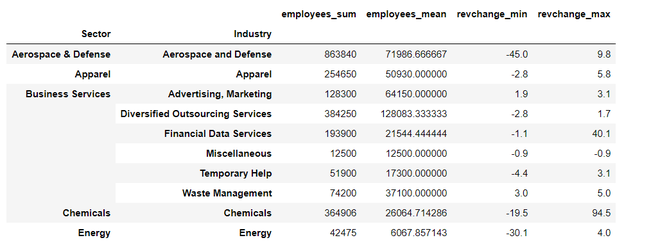Once the function is applied successfully all the columns are not flattened with the column name appended with the aggregate functions.

Full implementation:

## Python3

 `"""`` ``Program: For each "Sector" and "Industry" Find the total, average employees, and the minimum, maximum revenue change.  ``"""`` ` `import` `pandas as pd`` ` `"""`` ``Function: Convert hierarchial columns to non-hierarchial columns``   ``params: dataframe with hierarchial columns``  ``return : dataframe with non-hierarchial columns``"""`` ` ` ` `def` `return_non_hierarchial(df):``    ``df.columns ``=` `[``'_'``.join(x) ``for` `x ``in` `df.columns.to_flat_index()]``    ``return` `df`` ` ` ` `# load the dataset with rank as index``df ``=` `pd.read_csv(``    ``"https://raw.githubusercontent.com/sasankac/TestDataSet/master/Fortune500.csv"``, index_col``=``"Rank"``)`` ` `# remove unwanted columns``remove_columns ``=` `[``'Website'``, ``'Hqaddr'``, ``'Hqzip'``, ``'Hqtel'``, ``'Ceo'``,``                  ``'Ceo-title'``, ``'Address'``, ``'Ticker'``, ``'Prftchange'``,``                  ``'Assets'``, ``'Totshequity'``]`` ` `df ``=` `df.drop(columns``=``remove_columns, axis``=``1``)`` ` `# Identify the data as per the requirement``df_result ``=` `(df``             ``.groupby([``'Sector'``, ``'Industry'``])``             ``.agg({``'Employees'``: [``'sum'``, ``'mean'``],``                   ``'Revchange'``: [``'min'``, ``'max'``]})``             ``.astype(``int``)``             ``.pipe(return_non_hierarchial))`` ` `# print the data``df_result.head(``15``)`

Output: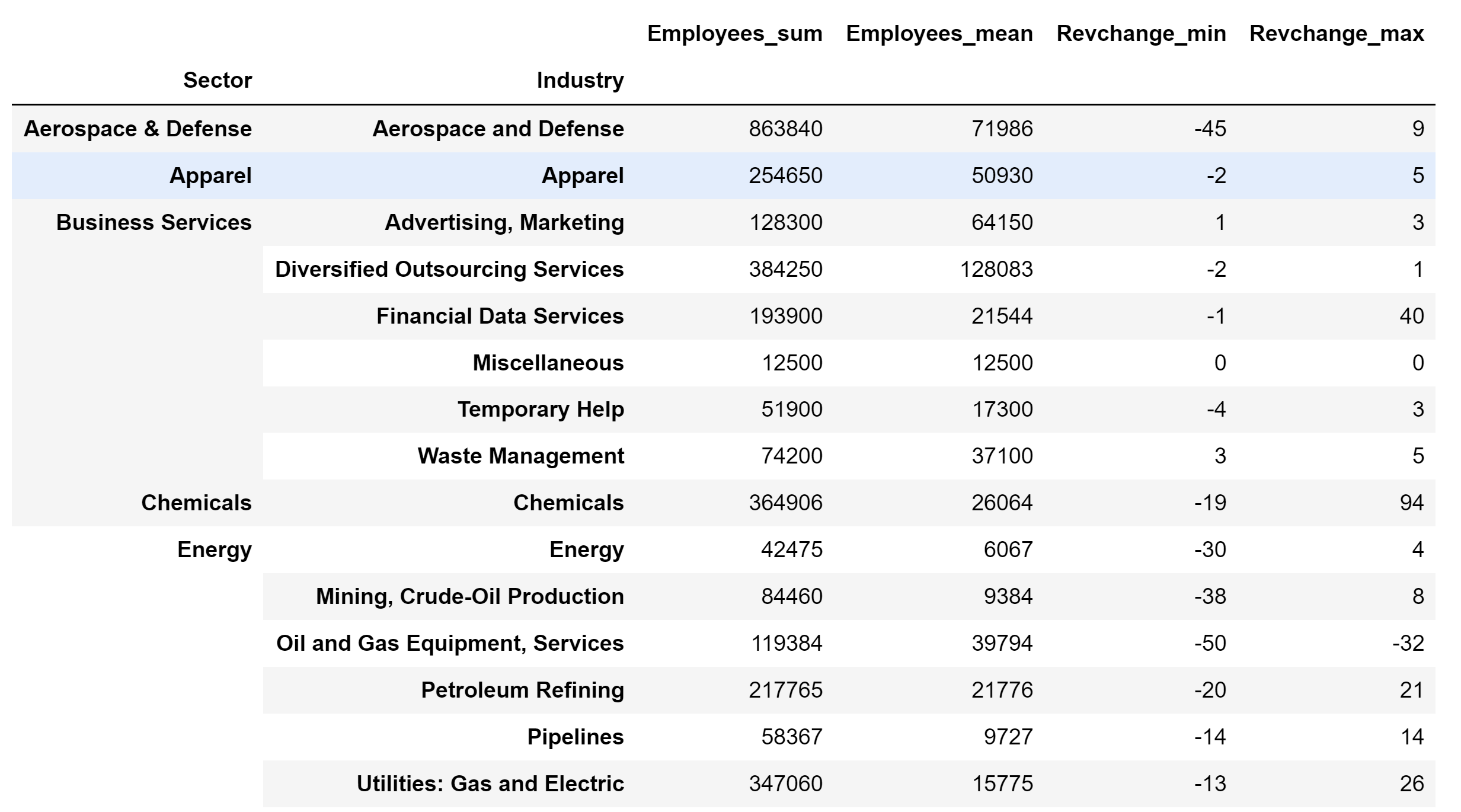Method 2:

Pandas have introduced named aggregation objects to create non-hierarchical columns. I will use the same requirement mentioned above and apply it to Named aggregation.

The syntax for this groupby method is as follows:

df.groupby([‘grouping column1′,’ grouping column2”]).agg({ ‘Named column’ = NamedAgg(column=’aggregate column’, aggfunc=’aggregate function’))

Implementation:

## Python3

 `"""`` ``Program: For each "Sector" and "Industry" Find the total, average employees, and the minimum, maximum revenue change.  ``"""`` ` `import` `pandas as pd`` ` `# load the dataset with rank as index``df ``=` `pd.read_csv(``    ``"https://raw.githubusercontent.com/sasankac/TestDataSet/master/Fortune500.csv"``, index_col``=``"Rank"``)`` ` `# remove unwanted columns``remove_columns ``=` `[``'Website'``, ``'Hqaddr'``, ``'Hqzip'``, ``'Hqtel'``, ``'Ceo'``,``                  ``'Ceo-title'``, ``'Address'``, ``'Ticker'``, ``'Prftchange'``,``                  ``'Assets'``, ``'Totshequity'``]`` ` `df ``=` `df.drop(columns``=``remove_columns, axis``=``1``)`` ` `# Identify the data as per the requirement``df_result ``=` `(df``             ``.groupby([``'Sector'``, ``'Industry'``])``             ``.agg(Employees_sum``=``pd.NamedAgg(column``=``'Employees'``, aggfunc``=``'sum'``),``                  ``Employees_average``=``pd.NamedAgg(``                      ``column``=``'Employees'``, aggfunc``=``'mean'``),``                  ``Revchange_minimum``=``pd.NamedAgg(``                      ``column``=``'Revchange'``, aggfunc``=``'min'``),``                  ``Revchange_maximum``=``pd.NamedAgg(column``=``'Revchange'``, aggfunc``=``'max'``))``             ``.astype(``int``))`` ` `# print the data``df_result.head(``15``)`

Output: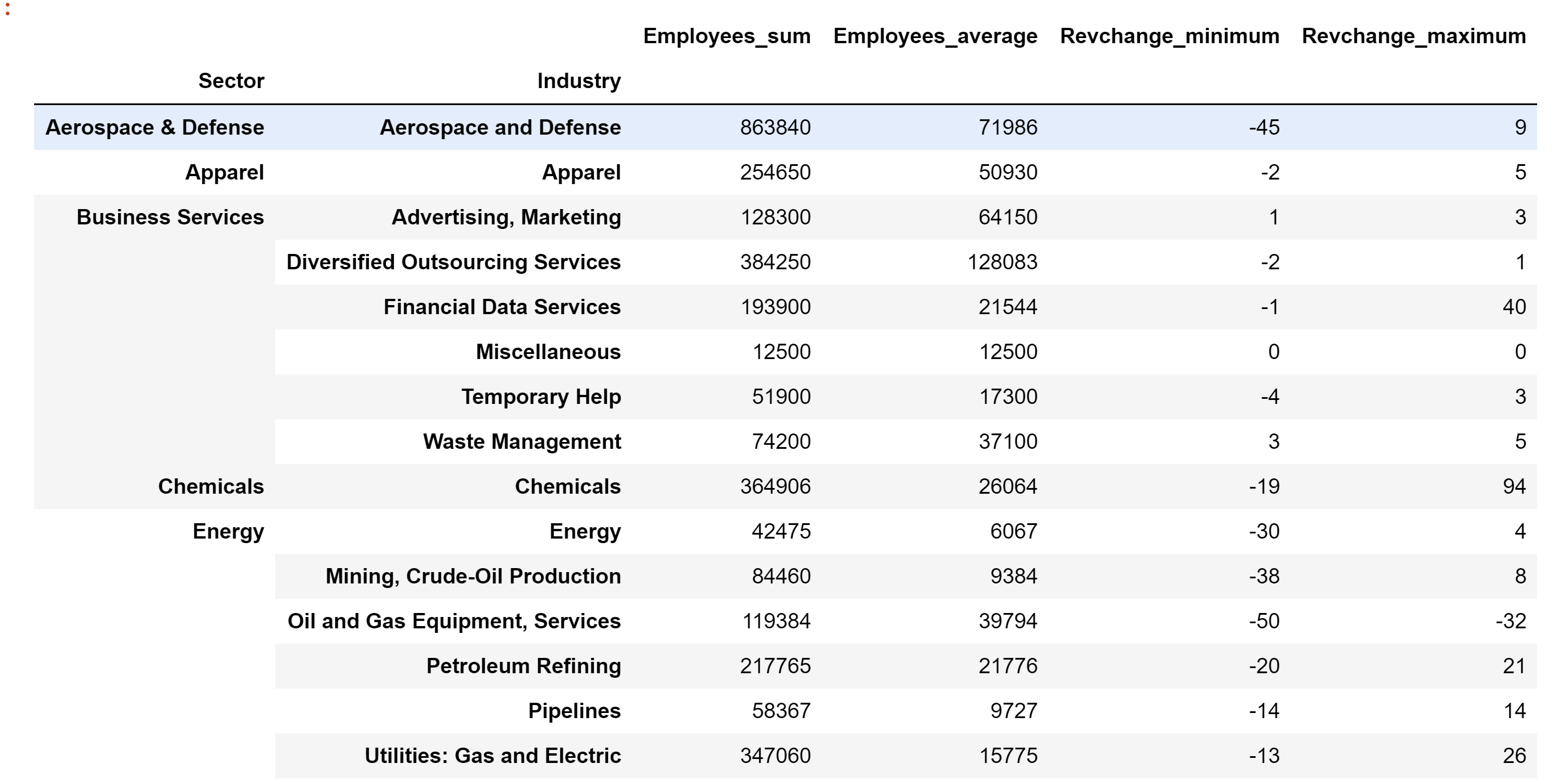Attention geek! Strengthen your foundations with the Python Programming Foundation Course and learn the basics.

To begin with, your interview preparations Enhance your Data Structures concepts with the Python DS Course. And to begin with your Machine Learning Journey, join the Machine Learning – Basic Level Course

My Personal Notes arrow_drop_up